# Legendre’s Ingenious Proof of Euler’s Polyhedron Formula

“The obvious way of doing things is not always the best way and it’s often a good idea to play around with patterns and push them beyond the bounds where you expect them to work. Because a little bit of insight and mathematics can go a very long way.”
Derek Muller

Euler’s polyhedron formula is often referred as The Second Most Beautiful Math Equation, second to none other than another identity (e^{iπ}+1=0) by The Mathematical Giant Euler. Today I’m going to write about this Polyhedron formula, and a beautiful proof of this formula, which was first discovered by another versatile mathematician Adrien-Marie Legendre.

Now, what is Euler’s Polyhedron Formula? What is it about?

Euler’s Polyhedron Formula basically gives us a fundamental and elegant result about Polyhedrons. In case you don’t know what is a polyhedron, the Greek suffix poly means many, and hedra means face. So roughly speaking, polyhedron is a three-dimensional shape that consists of multiple flat polygonal faces. The cover image of this blog shows some example of polyhedrons. Also, a Football (or a Soccer Ball if you prefer that name), is a polyhedron that contains 12 pentagonal face and 20 hexagonal face.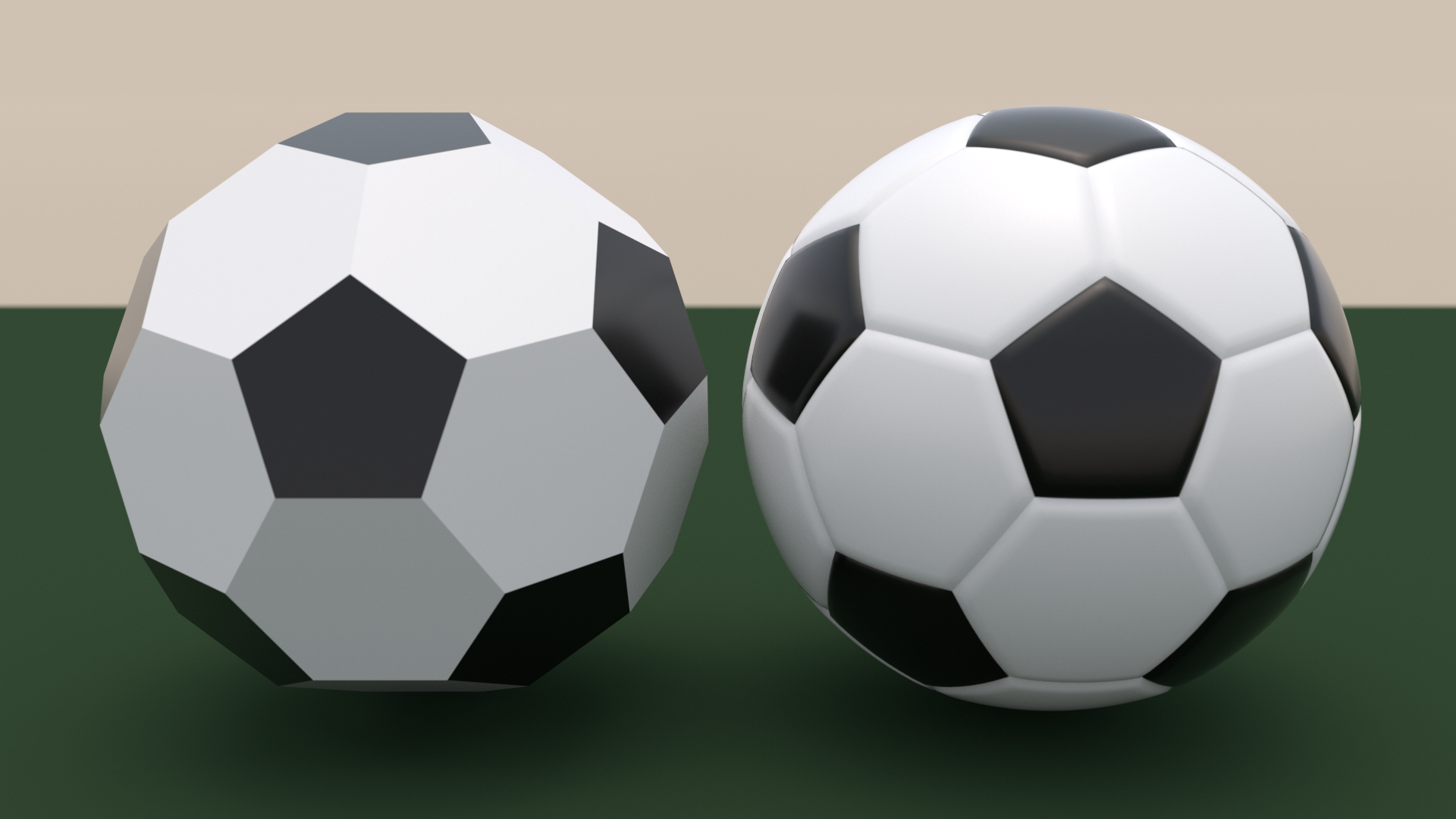Left: Polyhedral Football. Right: If you insert air into a polyhedral Football, the air pressure makes the flat polygonal faces a bit curvy. That’s why a Football looks more like a Sphere. (Imagine playing football with the sharp Polyhedral Football in the left xD)

Now, what does a Polyhedron have? By definition, a polyhedron has some polygonal Faces. Here the yellow triangles and red pentagons are the faces. Now, a face is polygon so by definitely it has some gon-s or sides. You can see here that sometimes two faces share some common side (that’s why it looks like a closed box, otherwise just a random collection of polygons wouldn’t be considered as polygons). These common sides are called Edges. In this example, the edges are marked grey. Also, three or more faces has some common endpoints. These common endpoints are called Vertices (plural of Vertex).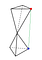Example of a non-convex Polyhedron. Here the green line segment through the red vertex and blue vertex does not lie on or inside the polyhedron. (Pardon my poor and low effort edit. I edited it using MS Paint -_- )

Now that we’ve learnt about Vertices, Edges, Faces; the next thing is Convex Polyhedron. The formal definition of convex polyhedron deals with convex hull, so I’m not gonna explain that now. If you’re interested, you can look it up. The definition of Convex Polyhedron I’m gonna state here is: If you take any two points on or inside the polyhedron, the line segment through that two points will be strictly on or inside the polyhedron. The examples we’ve seen till now, every single one of them was convex polyhedrons. I’ve added some examples of non-convex Polyhedrons below.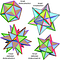Some Non-Convex Polyhedrons

Now we are on the same page, we know what is a Convex Polyhedron. Back to Euler’s formula. Euler’s formula establishes a relation between the number of Vertices, number of Edges, number of Faces in a convex Polyhedron. Let V, E, F respectively denotes the number of Vertices, Edges, Faces in a convex polyhedron. Then Euler’s formula states that

How simple a formula, yet so elegant and beautiful! :0 But to me, Legendre’s proof is somewhat more beautiful than the formula itself.

Okay, so how did Lagrange prove the formula?

Before diving into the proof, we need some background knowledge. First we need to know about Great Circles (Great Circles are often called Geodesic) and Geodesic Polygons. These are related to spherical geometry.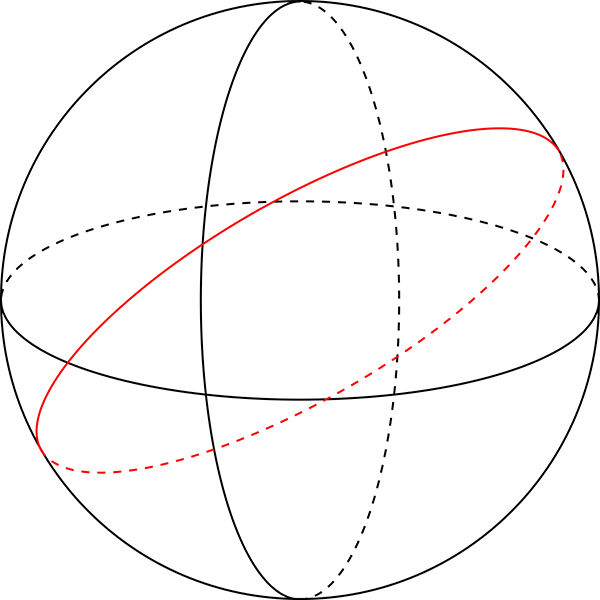Some Great Circles

So what is a Great Circle? Suppose we have a sphere. A Great Circle is any circle on the sphere that has the same radius as the sphere. For example, if we assume the Earth to be a perfect sphere, then the equator line is a great circle. Also, the lines of longitude are also great circles. Oftentimes, you may find the definition that great circle is the circle that cuts the sphere into two congruent hemispheres. That definition is equivalent to the definition I stated here.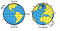Assuming the earth is a sphere, equator line and the lines of longitude are great circles. They are marked in this photo.

Now, about Geodesic Polygons, we shall define Geodesic Triangles first. Then we will expand the definition to higher polygons.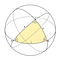Geodesic Triangle

A Geodesic Triangle is a region on the surface of the sphere, which is bounded by three geodesics (or great circles). Some books state it as Spherical Triangle too. Now, Euclidean Geometry insists that the sum of the angles in a Triangle must be 180 degrees, or π radian (throughout this blog, I shall prefer radians more than degrees; unless specified, the unit of angle is radian). That’s because of Eulid’s 5th Postulate. But this postulate doesn’t hold in spherical geometry. A Geodesic triangle can have angle sum more than 2π.

Wait, hold on a second. We know how to calculate angles between two lines. But the sides of a Geodesic Triangle are parts of some Geodesics. So how do we find the angle between two geodesics?

Tension not, my pal. We shall extend our notion of angle between two sides to angles between two intersecting circles.

Suppose two great circles intersect at some point B. Then we draw the tangents at point B to both of the circles. We know how to calculate angle between two lines, so we find out the angle between these two tangent lines. This angle is defined as the angle between those two circles.

Now a natural question arises, two circles can intersect each other at two points. Is the angle equal in both points? The answer is yes. The proof is left as an exercise for the reader.

Now, back to our point. As I said earlier, a Geodesic triangle can have angle sum more than π. For example, the larger triangle in the image below is a geodesic triangle with 3 right angles. :0

As you can guess, the angle sum of geodesic triangle is closely related to the area. Seems like, the larger the angle sum, the larger the area. This, in fact, is true. There is a theorem called Harriot-Girard Theorem that gives us a precise relation between sum of the angles and the area. The theorem states that: if a geodesic triangle on a unit sphere (sphere with radius 1) has angles a, b, c; then the area of that triangle will be a+b+c–π.

I’m not gonna show the rigorous proof of this theorem here. A visual demonstration is given in the Wolfram link above. Also a rigorous proof is given in the Princeton blog.

Now that we know what is Geodesic Triangle, can we extend the notion of triangle to any polygon? The answer is: yes, we can. In an analogous manner, we define a Geodesic Polygon as the area bounded by three or more geodesics. Needless to say, the sides of a Geodesic Polygon are parts of some great circles.

In this photo, ABCDE is a geodesic pentagon. The sides AB, BC, CD, DE, EA are actually parts of some geodesics.

In the same way as before, we can now generalize the Harriot-Girard Theorem for polygons. The generalized Harriot-Girard Theorem is: if an n-sided geodesic polygon has angles a₁, a₂, a₃, … , aₙ ; then the area of this polygon is: a₁ + a₂ + a₃ + … + aₙ –( n–2 )π = sum of angles–nπ+2π.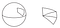Dividing an n-sided geodesic polygon into n-2 geodesic triangles

The proof to the generalization is just using the previous result. For any n-sided geodesic polygons, we can divide it into n-2 geodesic triangles. Just choose any vertex, then connect the other vertices to this chosen vertex using some great circles. Thus we obtain n-2 geodesic triangles. Notice that, the sum of the areas of these triangles is precisely the area of the polygon. Furthermore, the sum of all the angles of the triangles is equal to the sum of the angles of the polygon. Now, if we apply Harriot-Girard Theorem on all the n-2 triangles, we obtain the result.

Alright, enough about spherical geometry. How are these even related to our original problem, Euler’s Polyhedron Formula?

What Legendre does next is, he connects all these seemingly unrelated ideas from spherical geometry to solve a combinatorial problem.

Let’s consider a convex polyhedron with V vertices, E edges and F faces. Take any point X inside the polyhedron. Then construct a hollow sphere centered at X that surrounds the polyhedron completely. The units are not really important here. So we can assume without loss of generality that the sphere is a unit sphere.

What happens next is sheer magic! :0 We project the polyhedron onto the sphere through the point X. We can think of this projection as light and shadow. Assume that there is a lightbulb at the point X. Then we shall mark the shadows of the edges onto the sphere. The shadows will make some geodesic polygons.

The next trick Legendre pulls out of his sleeve is Counting in two ways. This means, if we calculate the same quantity in two different ways, the values we find using the two ways must be the same. Because we are essentially calculating the same thing. And how can a thing can have multiple different values?

What Legendre calculates here is the surface area of the sphere. One possible way to calculate surface area is: we know the formula surface area =4πr². Here the radius is 1, so the surface area is 4π.

We can calculate the same thing by adding the areas of the geodesic polygons we got after projecting. By the generalized Harriot-Girard Theorem, area=sum of angles–nπ+2π, where n is the number of edges of that geodesic polygon. Notice that, each face of the polyhedron corresponds to exactly one geodesic polygon. That means, there are total F polygons. If we take the sum of areas over all polygons, we get

If we choose a vertex of the polyhedron, the projected vertex makes some angles across some geodesic polygons. The sum of these angles is 2π (again, the proof is left as an exercise for the reader). (Σsum of angles) is basically sum of angles across all the vertices. So this quantity is 2πV.

Now, what was n? n was the number of edges in the polygon. From the definition of edge in a polyhedron, each edge is shared by exactly two faces. Hence, when we calculate Σn, we count each edge twice. So Σnπ = πΣn=π(2E)=2πE.

We showed earlier that there are total F polygons. And we are summing over these F polygons. So Σ2π=2πF. Σarea is the surface area of the sphere, which is 4π. Plugging in values, we get

Hence our desired formula is proved.

Now what’s so great about this proof? This proof connects seemingly unrelated concepts — spherical geometry, angles, areas— to prove a combinatorial theorem. The ideas are really very clever and beautiful. However, this proof suggests that Euler’s Polyhedron Formula is more than just a fundamental formula about polyhedrons, and there must be a close relation between this formula and geometry. And that indication eventually lead to the discovery of a new mathematical branch, Topology. :0

I started this with a quote, so it’s ideal to end with another quote. If you have any suggestions about new articles/improvements on this article/any questions, don’t hesitate to ask. You can comment here (I’m not really sure how commenting on Medium works), or email me, or send me a message on instagram, or send me a message on twitter, or send me a message on discord (atonu_#1514).

“A not good math paper has zero ideas, it’s just pushing through things that everybody already knows but nobody bothered to do. Then there are good math papers that have one new idea which is really shocking.”
- Alex Kontorovich

I like to think I’m x% of a philosopher and y% of an artist, where x is larger than y.

## More from Atonu Roy Chowdhury

I like to think I’m x% of a philosopher and y% of an artist, where x is larger than y.